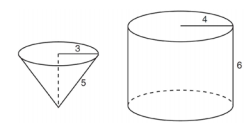mycollegehive
Determine and state the number of full cones of water needed to completely fill the cylinder with water.
JMAP geometry math cone0In the diagram below, a right circular cone with a radius of 3 inches has a slant height of 5 inches, and a right cylinder with a radius of 4 inches has a height of 6 inches.Determine and state the number of full cones of water needed to completely fill the cylinder with water.

41 viewsShareFollowUniversity of Lagos Nigeria
23 June 2020University of Benin Nigeria
23 June 20200Number of full cones of water needed to completely fill the cylinder with water, N = Volume of Cylinder, V1 / Volume of Cone, V2.

That is, N = V1/V2

V1 = πr12h1

where r1 and h1 are the radius and height of the cylinder respectively.

V1 = π(4)2(6)

= 96π

V2 = 1/3 πr22h2

Where r2 and h2 are radius and vertical height of the cone respectively.

From pythagorean's theorem, h2 = 52 - 32

h22 = 25 - 9

h22 = 16

Take square root of both sides

h2 = 4

Thus, V2 = 1/3 π(3)2(4)

= 12π

Therefore, N = 96π / 12π

= 8Share### Related Tags

JMAP

2 followers

141 questionsgeometry

2 followers

99 questionsmath

2 followers

988 questions